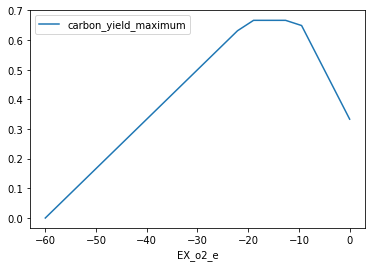# 6. Production envelopes¶

Production envelopes (aka phenotype phase planes) will show distinct phases of optimal growth with different use of two different substrates. For more information, see Edwards et al.

Cobrapy supports calculating these production envelopes and they can easily be plotted using your favorite plotting package. Here, we will make one for the “textbook” E. coli core model and demonstrate plotting using matplotlib.

:

import cobra.test
from cobra.flux_analysis import production_envelope

model = cobra.test.create_test_model("textbook")


We want to make a phenotype phase plane to evaluate uptakes of Glucose and Oxygen.

:

prod_env = production_envelope(model, ["EX_glc__D_e", "EX_o2_e"])

:

prod_env.head()

:

carbon_source carbon_yield_maximum carbon_yield_minimum flux_maximum flux_minimum mass_yield_maximum mass_yield_minimum EX_glc__D_e EX_o2_e
0 EX_glc__D_e 1.442300e-13 0.0 0.000000 0.0 NaN NaN -10.0 -60.000000
1 EX_glc__D_e 1.310050e+00 0.0 0.072244 0.0 NaN NaN -10.0 -56.842105
2 EX_glc__D_e 2.620100e+00 0.0 0.144488 0.0 NaN NaN -10.0 -53.684211
3 EX_glc__D_e 3.930150e+00 0.0 0.216732 0.0 NaN NaN -10.0 -50.526316
4 EX_glc__D_e 5.240200e+00 0.0 0.288975 0.0 NaN NaN -10.0 -47.368421

If we specify the carbon source, we can also get the carbon and mass yield. For example, temporarily setting the objective to produce acetate instead we could get production envelope as follows and pandas to quickly plot the results.

:

prod_env = production_envelope(
model, ["EX_o2_e"], objective="EX_ac_e", carbon_sources="EX_glc__D_e")

:

prod_env.head()

:

carbon_source carbon_yield_maximum carbon_yield_minimum flux_maximum flux_minimum mass_yield_maximum mass_yield_minimum EX_o2_e
0 EX_glc__D_e 2.385536e-15 0.0 0.000000 0.0 2.345496e-15 0.0 -60.000000
1 EX_glc__D_e 5.263158e-02 0.0 1.578947 0.0 5.174819e-02 0.0 -56.842105
2 EX_glc__D_e 1.052632e-01 0.0 3.157895 0.0 1.034964e-01 0.0 -53.684211
3 EX_glc__D_e 1.578947e-01 0.0 4.736842 0.0 1.552446e-01 0.0 -50.526316
4 EX_glc__D_e 2.105263e-01 0.0 6.315789 0.0 2.069927e-01 0.0 -47.368421
:

%matplotlib inline

:

prod_env.plot(
kind='line', x='EX_o2_e', y='carbon_yield_maximum');Previous versions of cobrapy included more tailored plots for phase planes which have now been dropped in order to improve maintainability and enhance the focus of cobrapy. Plotting for cobra models is intended for another package.